# Excel MIN function - usage and formula examples

This tutorial explains how to use the MIN function in Microsoft Excel 2007 - 2019, find the lowest value by a condition and highlight the bottom number in your range.

Today you will learn how to use basic but quite important MIN function in Excel. You'll see the ways to find the lowest value excluding zeroes, the absolute minimum and the smallest value based on some criteria. Furthermore, I'll show you the steps to highlight the least number and tell you what to do if your MIN function returns an error instead of the result.

Well, let's get started. :)

## Excel MIN function - syntax and examples

The MIN function checks your data range and returns the smallest value in this set.

The syntax for the MIN function is the following:

MIN(number1, [number2], …)

number1, [number2], … is the series of numbers from where you want to get a minimum. Number1 is required while [number2] and the following are optional.

There are up to 255 arguments allowed in one MIN formula. The arguments can be numbers, cells, arrays of references, and ranges. However, arguments like logical values, text, empty cells are ignored.

### How to use MIN in Excel - formula examples

The MIN formula is one of the easiest to apply in Excel. Let me prove it to you:

#### Example 1. Finding the smallest value

Let's say you have some fruits in stock. Your task is to check if you are running out of any. There are several ways to go:

Case 1: Enter each and every numeric value from the Qty in stock column into the formula:

`=MIN(366, 476, 398, 982, 354, 534, 408)`

Case 2: Reference the cells from the Qty column one by one:

`=MIN(B2,B3,B4,B5,B6,B7,B8)`

Case 3: Or simply reference the whole range of cells:

`=MIN(B2:B8)`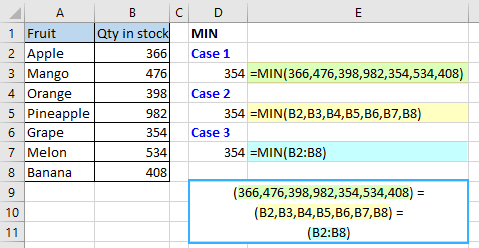Case 4: Alternatively, you can create a named range and use it instead to avoid any direct references:

`=MIN(Qty-in-stock)`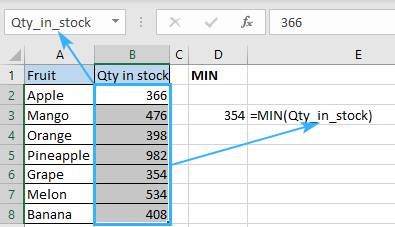#### Example 2. How to find the earliest date

Imagine that you are waiting for a few deliveries and would like to be ready for the most upcoming one. How to find out the earliest date in Excel? Easy! Use the MIN function following the same logic from example 1:

Apply MIN formula and select the dates either by referencing the cells directly:

`=MIN(B2:B8)`

Or the named range:

`=MIN(Delivery-date)`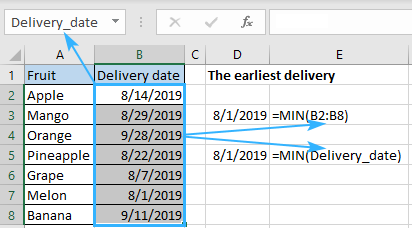#### Example 3. Looking for an absolute minimum

Supposing that you have a range of numbers and need to detect not simply the lowest but the absolute minimum there. The MIN alone won't be able to handle that since it will just look for the minimal number. Here you need a helper function that can convert all negative numbers to positive ones.

Is there a ready-made fix for this case? The question was rhetorical, there is a solution for any task in Excel. If you have any doubts, just look through our blog. :)

But let's get back to our task. The ready-made solution for this particular case is called ABS function which returns the absolute value of numbers you specify. Thus, the combination of MIN and ABS formulas will do the trick. Just enter the following formula in any blank cell:

`{=MIN(ABS(A1:E12))}`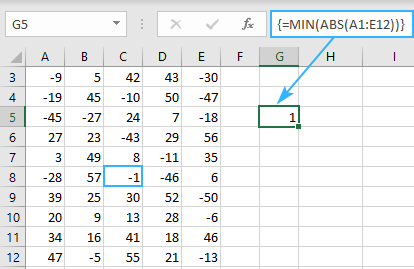Note! Did you notice the curly brackets around the formula? It's a sign that this is an array formula and it needs to be entered via Ctrl + Shift + Enter, not just Enter. You can read more about array formulas and their usage here.

## How to find the lowest value in Excel excluding zeros

Does it seem like you know everything about locating minimum values? Don't jump to conclusions, there is plenty left to learn. For example, how would you determine the least non-zero value? Any ideas? Don't cheat and google it, just keep reading ;)

The thing is, MIN formula works with not only positive and negative numbers but also with zeroes. If you don't want zeros to be that minimum, you need some help from the IF function. Once you add the limitation that your range should be more than zero, the expected result won't keep you waiting. Here is a sample of the formula to use:

`{=MIN(IF(B2:B15>0,B2:B15))}`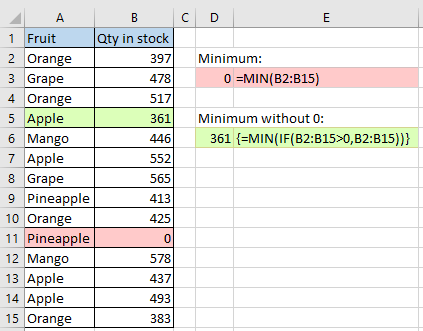You must have noticed the curly brackets around the array formula. Just remember that you don't enter them manually. They appear one you hit Ctrl + Shift + Enter on your keyboard.

## Finding the minimum based on a condition

Let's suppose you need to locate the least sales number of a specific fruit in a list. In other words, your task is to determine a minimum value based on some criteria. In Excel, conditions usually lead to using the IF function. All you need to do is make a perfect combination of MIN and IF to solve this task:

`{=MIN(IF(A2:A15=D2,B2:B15))}`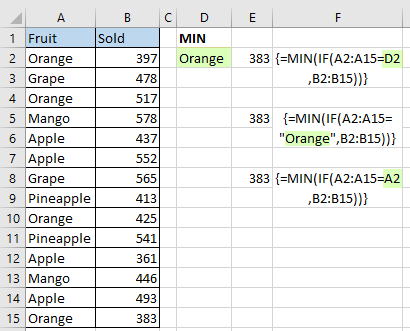Press Ctrl + Shift + Enter for this array function to work and enjoy.

Looks pretty easy, right? And how will you spot the minimum based on 2 or more conditions? How to find the minimum by multiple criteria? Maybe there is an easier formula for this task available in Excel? Please check this article to find it out. ;)

## Highlight the smallest number in Excel

And what if you don't need to return the smallest value is, but want to find it in your table? The easiest way to guide your eye to this cell is to highlight it. And the most straightforward way to do that is to apply conditional formatting. It's even simpler than creating a formula:

1. Create a new conditional formatting rule by clicking Conditional formatting -> New Rule
2. Once the New Formatting Rule dialog opens, select the “Format only top or bottom ranked values” rule type
3. Since the task is to find the one and only lowest value, choose the Bottom option from the drop-down list and set 1 as a quantity of cells to highlight.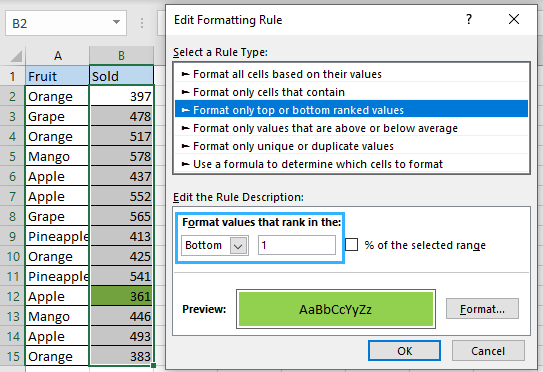But what to do if there is a zero in your table again? How to ignore zeroes when highlighting the bottom value? No worries, there is a trick for this case too:

1. Make a new conditional formatting rule selecting the “Use formula to determine which cells to format” option.
2. Enter the following formula in the Format values where this formula is true field: `=B2=MIN(IF(\$B\$2:\$B\$15>0,\$B\$2:\$B\$15))` Where B2 is the first cell of the range to find the lowest value in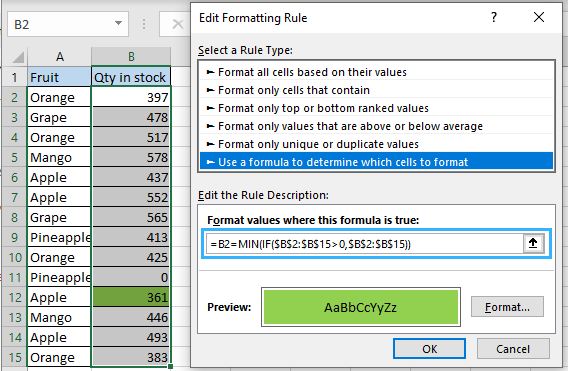3. Set the color (Edit Formatting rule -> Format… -> Fill) and hit OK.
4. Enjoy :)

## Why doesn't the MIN function work?

In the ideal world, all the formulas would work like a charm and return the correct results once you hit Enter. But in the world we live in it happens that formulas return error instead of the result we need. No worries, the error itself always hints at its possible cause. You just need to have a closer look at your formula.

#### Fixing the #VALUE error in MIN formula

Generally, you get the #VALUE! error message when at least one of the values used in a formula is incorrect. Regarding MIN, it may occur when one of the arguments is corrupted e.g. something is wrong with the cells the formula refers to.

For instance, #VALUE! may appear if one of the arguments is a cell with an error or there is a typo in the cell reference.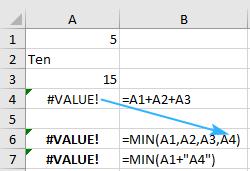#### What can cause the #NUM! error?

Excel shows #NUM! error when it is impossible to calculate your formula. It usually takes place when the numeric value is too big or small to be displayed in Excel. The allowed numbers are those between -2.2251E-308 and 2.2251E-308. If one of your arguments is outside this range, the function will return #NUM!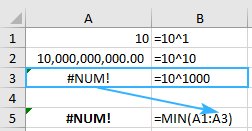#### I'm getting #DIV/0! error, what to do?

Fixing #DIV/0! is easy. Don't divide by zero! :) No kidding, this is the one and only solution for this issue. Check your data range for the cell with #DIV/0!, correct it and the formula will return the result right away.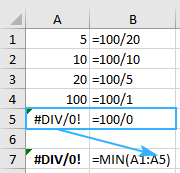#### Looking for a minimum but getting the #NAME? error?

#NAME? means that Excel can't recognize the formula or its arguments. The most possible reason for this result is a typo. You may either misspell the formula or put incorrect arguments to cause such result. Moreover, text representations of numbers will cause this error.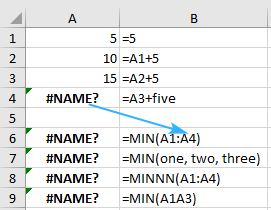The other possible cause of this problem lays in a named range. So, if you reference an unexisting range or there is a typo in it, you'll see #NAME? in the cell you're expecting your minimum to appear.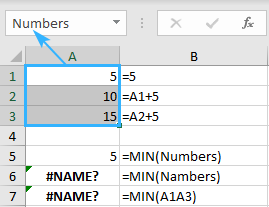These are the ways to find a minimum using the Excel MIN function. For you, I covered different approaches to find the lowest value in Excel and to locate the absolute minimum. You may consider this your cheat sheet and use it whenever you need to find the smallest number based on a condition and to prevent and fix the possible errors.

That's it for today. Thank you for reading! Please feel free to share your thoughts and questions in the comments section, I'll be glad to get feedback from you! :)

## You may also be interested in:

60+ professional tools for Excel